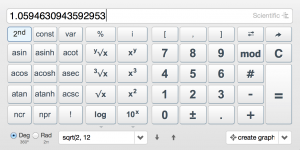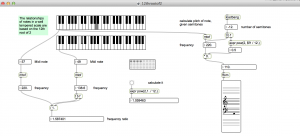# 12th root of 2

The note relationships in a chromatic scale are based on the 12th root of 2.

An octave has a ratio of 2. An octave is divided into 12 equal steps.

For example, to find the ratio of one semi-tone (half step), on a scientific calculator, use this button:Here’s the result where x = 2 and y = 12In a computer program, you could use the pow() function calculate 2 to the 1/12 power. Here’s an example in javascript:This Max patch shows the relationships between any two notes and how to calculate the pitch of a note based on the semi-tone interval. You might use it as a starting point to design your own scales. For example, a 13 note chromatic scale.  Or relationships based on randomness.